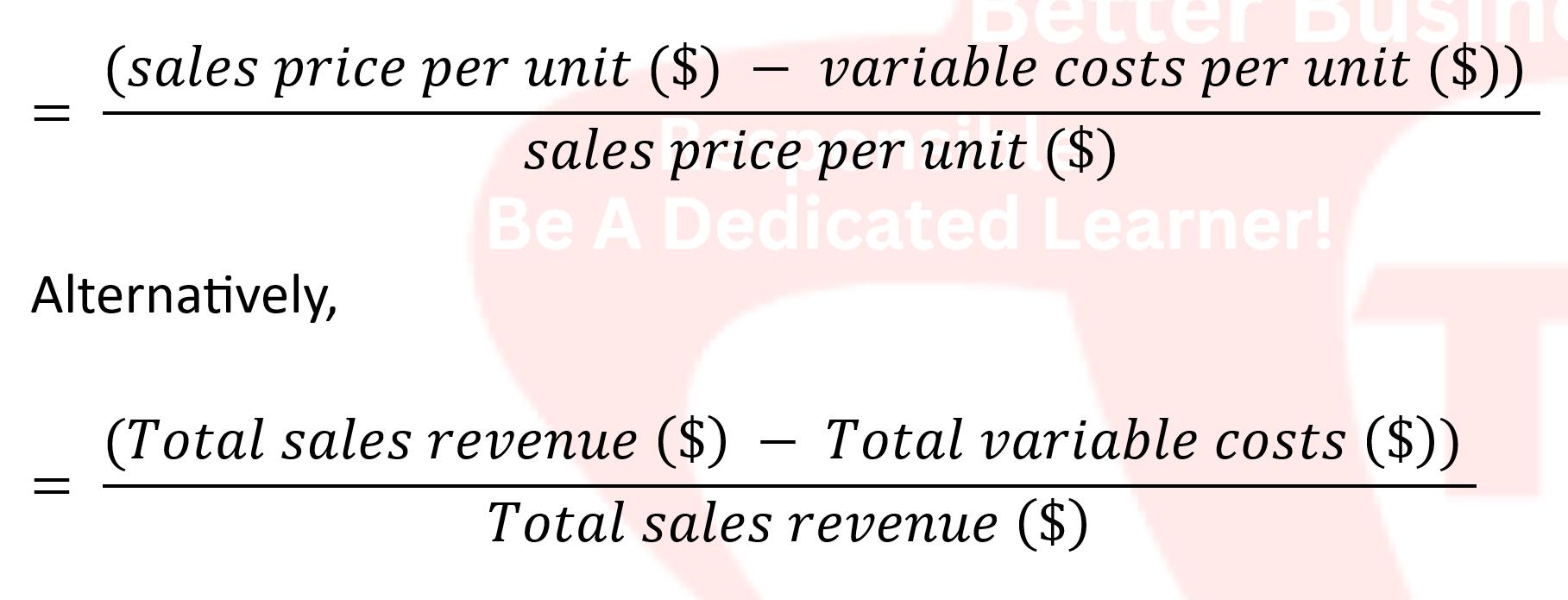# 【A.07.00】What is the C/S ratio?

Briefing Note
Reading comprehension is a skill that empowers lifelong learning intelligence for a better self.
The contribution to sales ratio..

✅ ..also known as the contribution margin ratio.

✅ ..a financial metric used in a business to evaluate the profitability of a product or service.

✅ ..measures the percentage of each sales dollar that is available to contribute towards covering fixed costs and generating profit after accounting for variable costs.

✅ ..by dividing the contribution margin by the sales revenue.✅ The contribution margin is calculated as the difference between the sales revenue and variable costs.

🚩 Contribution margin per unit (\$) = sales price per unit (\$) — variable costs per unit (\$)

Alternatively,

🚩 Total contribution margin (\$) = Total sales revenue (\$) — Total variable costs (\$)

✅ ..Mathematically, the contribution-to-sales ratio can be expressed as:✅ ..let’s say a company generates \$100,000 in sales revenue and incurs \$60,000 in variable costs,

🚩 the contribution margin would be (\$100,000 — \$60,000) = \$40,000 ; and

🚩 the contribution to sales ratio would be (\$40,000 ➗ \$100,000) = 0.4 or 40%

✅ ..for managers to analyse the profitability of products or services and to make decisions about pricing, production levels, and cost management.